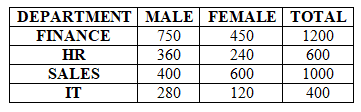# IBPS PO Prelims Quantitative Aptitude Questions 2021 (Day-04)

Dear Aspirants, Our IBPS Guide team is providing new series of Quantitative Aptitude Questions for IBPS PO Prelims 2021 so the aspirants can practice it on a daily basis. These questions are framed by our skilled experts after understanding your needs thoroughly. Aspirants can practice these new series questions daily to familiarize with the exact exam pattern and make your preparation effective.

Start Quiz

Caselet

Directions (01-05): Study the following information carefully and answer the questions given below.

There are total 3200 employees in the company. The number of HR in the company is 40% less than the number of sales employees. Ratio of the number of male to female employees in IT department 7:3 and the total number of female employees in the company is 1410. Total number of Finance employees is 1200 and the ratio of the total number of employees in IT to finance is 1:3. The number of female employees in Sales department is 50% more than the number of male employees in sales department. Total number of female employees in HR department is 240.

1) What is the difference between the total number of employees in IT and the number of female employees in Sales?

A.100

B.160

C.200

D.240

E.300

2) What is the ratio of the average of number of male employees in HR and sales together to the average number of female employees in Finance and IT together?

A.76:59

B.76:57

C.73:57

D.71:56

E.None of these

3) The number of male employees in Finance is what percent of the total number of employees in Sales department?

A.45%

B.55%

C.65%

D.75%

E.80%

4) Total number of employees in HR department is what percent of the total number of employees in the company?

A.12.5%

B.15.75%

C.18.75%

D.20.50%

E.None of these

5) What is the ratio of the total number of employees in Finance to Sales?

A.4:3

B.5:4

C.2:1

D.6:5

E.None of these

Mixture and allegation

6) The vessel contains 45 liters of milk and water in the ratio of 5:4. If x liters of water is added to the mixture and the ratio of the water and milk ratio becomes 5:4, then find the value of x?

A.12.5 liters

B.13.5 liters

C.11.5 liters

D.10.5 liters

E.None of these

Average

7) If the average price of 4 pen and 6 eraser is Rs.22.8 and the average price of 7 pen and 8 eraser is Rs.23.6, then find the price of 3 eraser?

A.Rs.36

B.Rs.54

C.Rs.60

D.Rs.48

E.None of these

percentage

8) Rahul scored 45% of the marks in physics and Rohit scored 108 marks in Physics. If the maximum mark in Physics is 120, then what is the average mark scored by Rahul and Rohit?

A.78

B.81

C.84

D.88

E.75

Percentage

9) The population growth of Chennai increases every year by 10% and the number of population in 2018 is 89540, what is the population in Chennai in 2016?

A.74000

B.76000

C.78000

D.72000

E.70000

Probability

10) A box contains 6 apples and 4 oranges. If 4 fruits are drawn at random from the box, then what is the probability that three is apple and one is orange?

A.2/35

B.3/35

C.4/35

D.1/7

E.None of these

Directions (1-5) :

Total number of employees = 3200

Number of female employees = 1410

Number of male employees = 3200 – 1410 = 1790

Total number of Finance employees = 1200

Total number of IT employees = 1/3 * 1200 = 400

Number of male IT employees = 400 * 7/10 = 280

Number of female IT employees = 400 * 3/10 = 120

Total number of female HR employees = 240

Total number of employees in HR and Sales = 3200 – 1200 – 400 = 1600

Number of employees in sales = x

Number of employees in HR = x * 60/100 = 3x/5

x + 3x/5 = 1600

8x = 1600 * 5

x = 1000

Number of employees in HR = 3 * 1000/5 = 600

Number of male employees in sales = a

Number of female employees in Sales = a * 150/100 = 3a/2

a + 3a/2 = 1000

5a = 2000

a = 400

Number of female employees in sales = 1200/2 = 600

Number of male employees in HR = 600 – 240 = 360

Number of female employees in Finance = 1410 – 240 – 600 – 120 = 450

Number of male employees in Finance = 1200 – 450 = 750Difference = 600 – 400 = 200

Required ratio = ((360 + 400)/2):((450 + 120)/2)

= 380:285

= 76:57

Required percentage = 750/1000 * 100 = 75%

Required percentage = 600/3200 * 100 = 18.75%

Required ratio = 1200:1000

= 6:5

Milk = 5/9 * 45 = 25 liters

Water = 4/9 * 45 = 20 liters

25/(20 + x) = 4/5

80 + 4x = 25 * 5

4x = 45

x = 11.25 liters

4P + 6E = 228 —-(1)

7P + 8E = 23.6 * 15 —(2)

(1) * 4 – (2) * 3

16P – 21P = -150

P = 30

6E = 228 – 120

E = 18

Required price = 18 * 3 = Rs.54

Marks scored by Rahul = 45/100 * 120 = 54

Required average = (54 + 108)/2 = 81

Population in 2016 = x

x * 110/100 * 110/100 = 89540

x = 74000